# python tutorial - Python Program For Armstrong Number - learn python - python programming

## Armstrong Number

• If the given number is equal to the sum of the Nth power of each digit present in that integer then, that number can be Armstrong Number. For example 370 is Armstrong Number.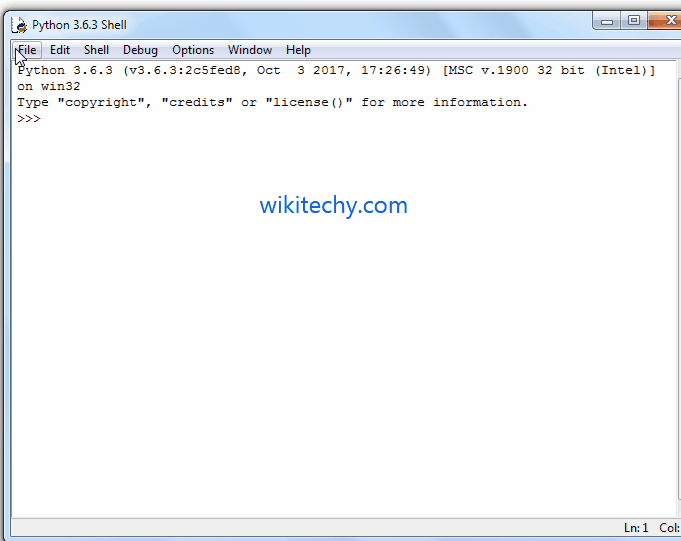Learn Python - Python tutorial - Python Program For Armstrong Number - Python examples - Python programs

Number of individual digits in 370 = 3
370 = 3³ + 7³ + 0³
= 27 + 343 + 0 = 370

• Below steps will show you the common approach to check for the Armstrong Number

## Steps:

• User has to enter any number
• Counting the Number of individual digits (For Example, 370 means 3)
• Divide the given number into individual digits (For Example, Divide 370 into 3, 7 and 0)
• Calculate the power of n for each individual and add those numbers
• Compare original value with Sum value.
• If they exactly matched then it is Armstrong number else it is not Armstrong
• To Write Python Program For Armstrong Number Using While Loop, For Loop, Functions and Recursion. We will also show you, Armstrong Numbers between 1 to n.

## Python Program for Armstrong Number Using While Loop

• This Python program allows the user to enter any positive integer and then, this program will check whether a number is Armstrong Number or Not using the Python While Loop

## Output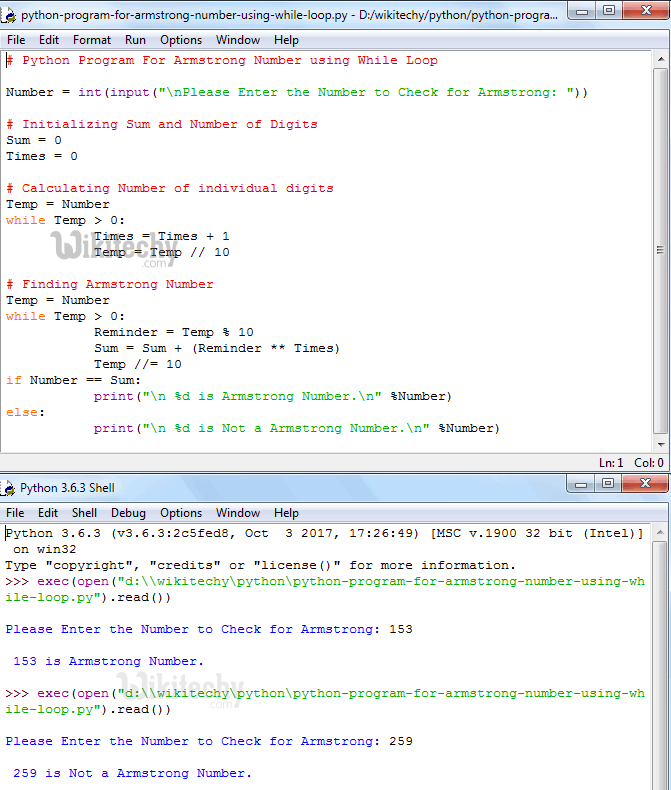Learn Python - Python tutorial - Python Program for Armstrong Number Using While Loop - Python examples - Python programs

## Analysis

• This program allows the user to enter any positive integer and then, that number is assigned to variable Number.
• Next, We assign the original value to the Temp variable. This will help us to preserve our original value and then do all the manipulation on Temp variable.
• Below While loop will make sure that, the given number is greater than 0. Statements inside the while loop will split the numbers and counts number of individual digits inside the given number.
• Second While loop will make sure that, the given number is greater than 0. Let us see the working principle of this while loop in iteration wise

User Entered value: Number = 9474 and Sum = 0
Temp = Number
Temp = 9474

## First Iteration

Reminder = Temp %10
Reminder = 9474 % 10 = 4
Sum = Sum + pow (Reminder, Times)
For this example, Times =4 because number of digits in 9474 = 4. So,
Sum = Sum + (Reminder * Reminder * Reminder * Reminder)
Sum = 0 + (4 * 4 * 4 * 4)
Sum = 0 + 256
Sum = 256
Temp = Temp /10
Temp = 9474 /10
Temp = 947

NOTE: If the number of digits count is 5 then Reminder will be multiplied by 5 times.

## Second Iteration

From the first Iteration the values of both Temp and Sum has been changed as: Temp = 163 and Sum = 256
Reminder = Temp %10
Reminder = 947 % 10 = 7
Sum = Sum + (Reminder * Reminder * Reminder * Reminder)
Sum = 256 + (7 * 7 * 7 * 7)
Sum = 256 + 2401
Sum = 2657
Temp = Temp /10
Temp = 163 /10
Temp = 94

## Third Iteration

From the Third Iteration the values of both Temp and Sum has been changed as:
Temp = 94 and Sum = 2657
Reminder = Temp %10
Reminder = 94 % 10 = 4
Sum = Sum + (Reminder * Reminder * Reminder * Reminder)
Sum = 2657 + (4 * 4 * 4 * 4)
Sum = 2657 + 256
Sum = 2913
Temp = Temp /10
Temp = 94 /10
Temp = 9

## Fourth Iteration

From the Fourth Iteration the values of both Temp and Sum has been changed as:
Temp = 9 and Sum = 2913
Reminder = Temp %10
Reminder = 9 % 10 = 0
Sum = Sum + (Reminder * Reminder * Reminder * Reminder)
Sum = 2913 + (9 * 9 * 9 * 9)
Sum = 2913 + 6561
Sum = 9474
Temp = Temp /10
Temp = 9/10
Temp = 0

• Here Number = 0 so, the while loop condition will fail if ( Number == Sum ) – Condition will check whether the user enter number is exactly equal to Sum number or not. If this condition is True, then it is Armstrong else the given number is not Armstrong number
• if ( Number == Sum )
• if(9474 == 9474) –TRUE, Means Armstrong

NOTE: If you are finding the Armstrong number below 1000 then you can simply remove the while loop to count the number of digits in a number and then replace the below code

## Python Program for Armstrong Number Using For Loop

• This Python program allows the user to enter any positive integer and then, this program will check whether a number is Armstrong Number or Not using Python For Loop

## Output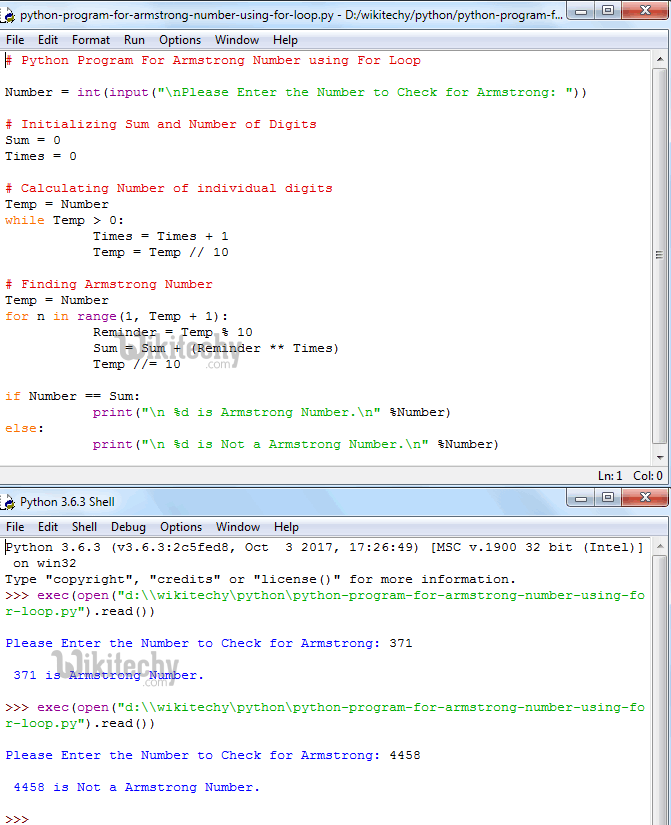Learn Python - Python tutorial - Python Program for Armstrong Number Using For Loop - Python examples - Python programs

• We just replaced the While loop in the above example with the For loop.

## Python Program for Armstrong Number Using Functions

• This Python program allows the user to enter any positive integer and then, this program will check whether a number is Armstrong Number or Not using Functions

## Output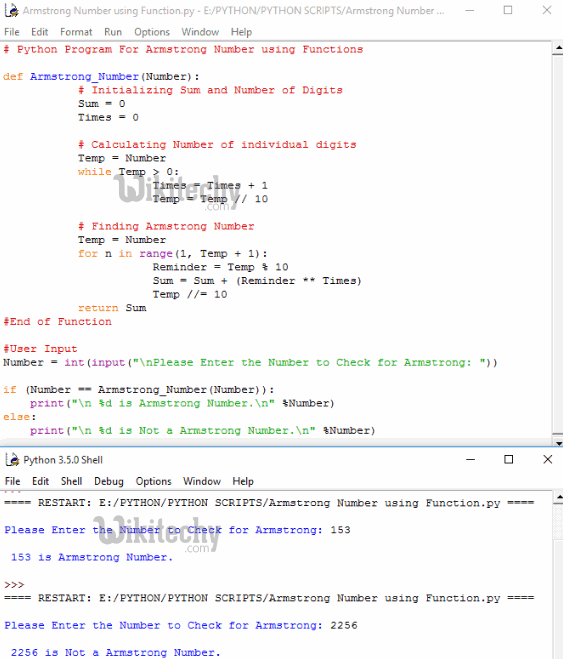Learn Python - Python tutorial - Python Program for Armstrong Number Using Functions - Python examples - Python programs

## Analysis

• In this example we defined following function to perform all the necessary calculations and return Sum
• When the compiler reaches to following code inside If statement then, the compiler will immediately jump to above specified function

## Python Program for Armstrong Number Using Recursion

• This Python program allows the user to enter any positive integer and then, this program will check whether a number is Armstrong Number or Not using Recursion concept.

## Output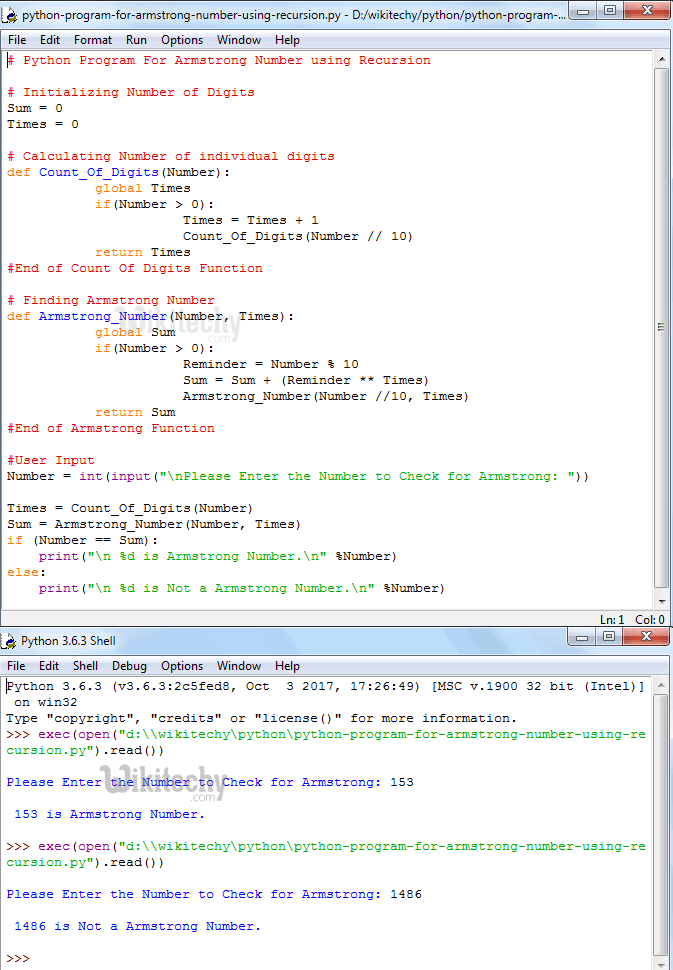Learn Python - Python tutorial - Python Program for Armstrong Number Using Recursion - Python examples - Python programs

## Analysis

• In this example we defined two recursive functions. Following function will accept integer values as parameter value and count the number of individual digits in a number recursively.
• Following function will accept two integer values as parameter values and will perform all the necessary calculations and return Sum
• Following Statement will help to call the function Recursively with updated value. If you miss this statement then, after completing the first line it will terminate. For example,

Number = 153
Then the Output will be 27

• Lets see the If statement inside the above specified functions
• if (Number > 0) will check whether the number is greater than 0 or not. For Recursive functions it is very important to place a condition before using the function recursively otherwise, we will end up in infinite execution (Same like infinite Loop).

## Python Program to Find Armstrong Numbers between the 1 to n

• This Python program allows the user to enter minimum and maximum values. This program will find the Armstrong Numbers between the Minimum and Maximum values.

## Output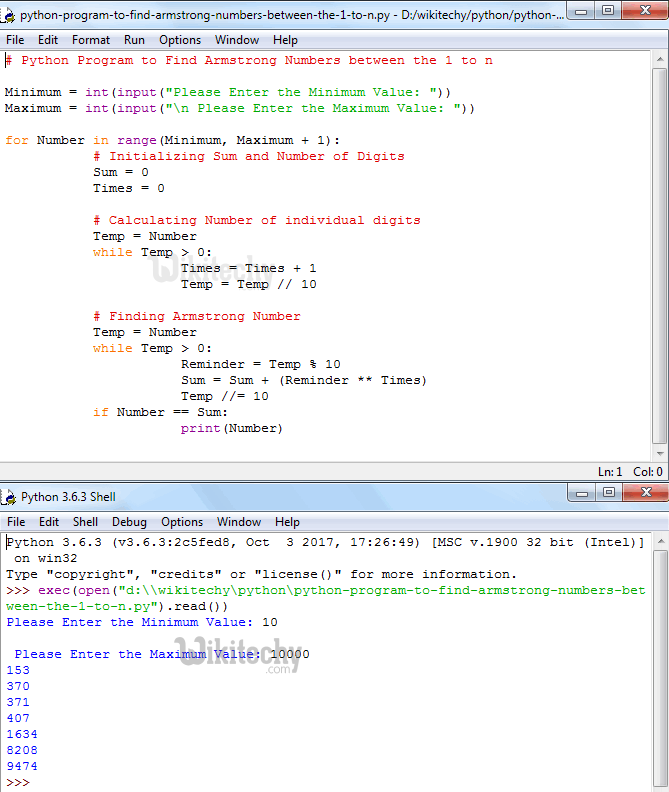Learn Python - Python tutorial - Python Program to Find Armstrong Numbers between the 1 to n - Python examples - Python programs

## Analysis:

• Following statements present in this program allows the user to enter minimum and maximum values.
• This For Loop helps compiler to iterate between Minimum and Maximum Variables, iteration starts at the Minimum and then it will not exceed Maximum variable.
• if(Number == Sum) -– condition will check whether the sum of the power N for each digit present in that integer is equal to given number or not. If this condition is True, then it is Armstrong else the given number is not Armstrong number.
• If this condition is True then, below statement will be printed